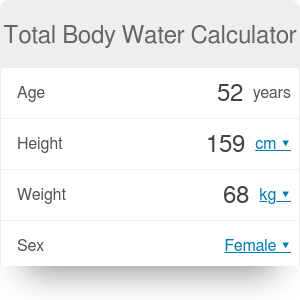Age
years
Height
ft
Weight
lb
Sex
female
Total body water
liters
Percentage of body weight
%

# Total Body Water Calculator

By Małgorzata Koperska, MD

This total body water calculator uses your age, height, weight, and sex to estimate the volume of water in your body. It is based on a formula developed by dr. P.E. Watson and his team and described in 1980 in the article Total body water volumes for adult males and females estimated from simple anthropometric measurements. If you read on, you can understand the formula and see why calculating body water by weight wouldn't be accurate.

## How does total body water calculator work?

Our calculator uses dr. P.E. Watson's formula:

• For males:
`TBW = 2.447 - 0.09156 * age + 0.1074 * height (cm) + 0.3362 * weight (kg)`
• For females:
`TBW = -2.097 + 0.1069 * height (cm) + 0.2466 * weight (kg)`

According to the article, these equations calculate the total body water for adults of any age.

## Why is the total body water calculated by body weight, height, age, and sex?

You can get a rough estimate of the total body water volume on the assumption that the average human adult male consists of approximately 60% water and the average adult female is approximately 50% of water. However, compared to several other advanced methods, the formula developed by dr. P.E. Watson gave the best overall results.

## How to interpret the calculated body water percentage?

The normal total body water volume range is 58 ± 8% for males and 48 ± 6% for females.

The body water is broken down into the following compartments:

• Intracellular fluid (2/3 of body water) is the fluid contained within cells.
• Extracellular fluid (1/3 of body water) is the fluid contained in areas outside of cells:
• Plasma (1/5 of extracellular fluid), which is a part of total blood volume;
• Interstitial fluid (4/5 of extracellular fluid);
• Transcellular fluid (a.k.a. "third space," normally ignored in calculations) contained inside organs, such as the gastrointestinal, cerebrospinal, peritoneal, and ocular fluids.
Małgorzata Koperska, MD

## Get the widget!

Total Body Water Calculator can be embedded on your website to enrich the content you wrote and make it easier for your visitors to understand your message.

It is free, awesome and will keep people coming back!